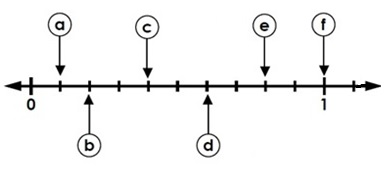# Reading Decimal Position on a Number Line: Tenths Online Quiz

Following quiz provides Multiple Choice Questions (MCQs) related to Reading Decimal Position on a Number Line: Tenths. You will have to read all the given answers and click over the correct answer. If you are not sure about the answer then you can check the answer using Show Answer button. You can use Next Quiz button to check new set of questions in the quiz.Q 1 - The letter b on the number line represents the decimalA - 2.0

B - 0.2

C - 0.1

D - 2

### Explanation

The number line is divided into tenths between 0 and 1

The letter b on the number line points to the decimal two tenths or 0.2

Q 2 - The letter h on the number line represents the decimalA - 0.4

B - 1.3

C - 1.4

D - 1.5

### Explanation

The number line is divided into tenths between 1 and 2

The letter h on the number line points to the decimal one and four tenths or 1.4

Q 3 - The letter e on the number line represents the decimalA - 0.8

B - 8.0

C - 1.8

D - 8

### Explanation

The number line is divided into tenths between 0 and 1

The letter e on the number line points to the decimal eight tenths or 0.8

Q 4 - The letter j on the number line represents the decimalA - 0.8

B - 8.0

C - 1.7

D - 1.8

### Explanation

The number line is divided into tenths between 1 and 2

The letter j on the number line points to the decimal one and eight tenths or 1.8

Q 5 - The letter c on the number line represents the decimalA - 0.4

B - 4.0

C - 0.3

D - 0.5

### Explanation

The number line is divided into tenths between 0 and 1

The letter c on the number line points to the decimal four tenths or 0.4

Q 6 - The letter a on the number line represents the decimalA - 1.0

B - 0.1

C - 0.01

D - 0.2

### Explanation

The number line is divided into tenths between 0 and 1

The letter a on the number line points to the decimal one tenths or 0.1

Q 7 - The letter g on the number line represents the decimalA - 2.2

B - 0.2

C - 1.2

D - 1.1

### Explanation

The number line is divided into tenths between 1 and 2

The letter g on the number line points to the decimal one and two tenths or 1.2

Q 8 - The letter d on the number line represents the decimalA - 6.0

B - 0.5

C - 0.06

D - 0.6

### Explanation

The number line is divided into tenths between 0 and 1

The letter d on the number line points to the decimal six tenths or 0.6

Q 9 - The letter i on the number line represents the decimalA - 0.7

B - 1.07

C - 1.7

D - 0.07

### Explanation

The number line is divided into tenths between 1 and 2

The letter i on the number line points to the decimal one and seven tenths or 1.7

Q 10 - The letter f on the number line represents the decimalA - 1.0

B - 0.1

C - 10.0

D - 0.01

### Explanation

The number line is divided into tenths between 0 and 1

The letter f on the number line points to the decimal ten tenths or 1.0